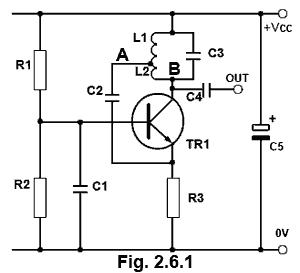# RF Oscillators Quiz

Try our quiz, based on the information you can find in Oscillators Module 2. Submit your answers and see how many you get right. If you get any answers wrong. Just follow the hints to find the right answer and learn about Oscillators as you go.

### 1.

Refer to Fig. 2.6.1. Which of the following component combinations are responsible for providing Sliding Class C bias?### 2.

Refer to Fig. 2.6.1. A typical frequency of oscillation for this circuit would be most likely to be in which of the following ranges?

### 3.

Which formula in Fig 2.6.2 should be used for finding the frequency of oscillation of a LC oscillator?### 4.

Refer to Fig. 2.6.1. What will be phase relationship between the waveforms at points A and B?

### 5.

Refer to Fig. 2.6.3. Which of the following governs the proportion of signal used as positive feedback?

### 6.

The output of a LC oscillator is often fed into a common collector amplifier stage. The reason for this is:

### 7.

Refer to Fig. 2.6.3. Which of the following actions would increase the frequency of the oscillator?### 8.

Refer to Fig. 2.6.4. What is the purpose of L1?### 9.

A typical specification for a quartz crystal oscillator would indicate a Q factor of:

### 10.

Refer to Fig. 2.6.5. In which of the following modes is the quartz crystal X1 operating?Top of Page